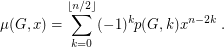# Matching polynomials of vertex transitive graphs (Solved)

 Importance: Medium ✭✭
 Author(s): Mohar, Bojan
 Subject: Graph Theory » Algebraic Graph Theory
 Keywords: matching polynomial vertex-transitive
 Posted by: Robert Samal on: April 12th, 2007
 Solved by: Cheng Yeaw Ku and William Chen (see [K])
Conjecture   For every integerthere exists a vertex transitive graphwhose matching polynomial has a root of multiplicity at least.

Letbe a graph of order. Denote bythe number of-matchings in. The matching polynomial ofis defined asIt is known that every matching polynomial has only real roots. See [HL, GG].

It would be interesting to solve the Conjecture even for, that is to find a vertex transitive graph whose matching polynomial has a nonsimple root. Such a graph would not have a hamiltonian path (see [HL, GG]), thus giving a negative answer to a problem of Lovász.

This conjecture is false.

Definition   Given a graphand a rootof, a vertexofis said to be-essential if the multiplicity ofas a root ofis one less than the multiplicity ofas a root of.

In [K], Ku and Chen prove the following analogue of Gallai's Lemma.

Theorem   Letbe a graph andbe a root of. If all vertices ofare-essential, thenhas multiplicity 1 as a root of.

Since every graph contains a-essential vertex, all vertices of a vertex-transitive graph are-essential. Thus the matchings polynomial of any vertex-transitive graph has only simple roots.

## Bibliography

[HL] O. J. Heilmann, E. H. Lieb, Theory of monomer-dimer systems, Comm. Math. Phys. 25 (1972), 190-232. MathSciNet

[GG] C. D. Godsil, I. Gutman, On the theory of the matching polynomial, J. Graph Theory 5 (1981), 137-144. MathSciNet

[K] Cheng Yeaw Ku and William Chen, An Analogue of the Gallai-Edmunds Structure Theorem for Nonzero Roots of the Matchings Polynomial (June 2008). Preprint available from arXiv

[M] B. Mohar, Problem of the Month

* indicates original appearance(s) of problem.